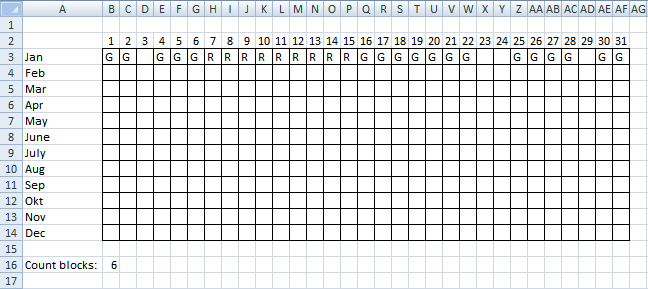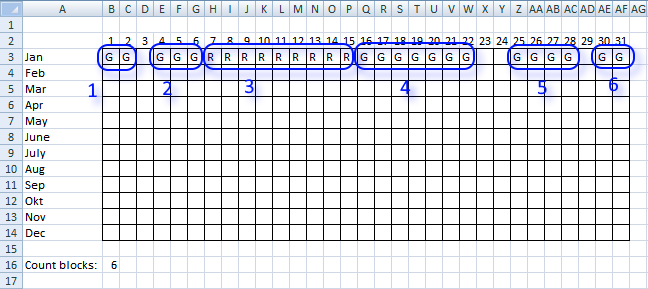Author: Oscar Cronquist Article last updated on December 24, 2018Question:

Is there a formula that can count blocks
For eg in your picture (see picture above) if the green blocks had the letter G and the Red blocks had the letter R and I had to return 4 as the answer -3G + 1R
Is this possible through a formula?

You can find the question here:
Count overlapping dates in excelThe image above shows the groups in row 3, the formula in cell B16 counts the number of groups.

Formula in B16:

=SUMPRODUCT(--(\$B\$3:\$AE\$3<>\$C\$3:\$AF\$3), --(\$C\$3:\$AF\$3<>""))+(\$B\$3<>"") + ENTER

### Explaining formula in cell B16

=SUMPRODUCT(--(\$B\$3:\$AE\$3<>\$C\$3:\$AF\$3), --(\$C\$3:\$AF\$3<>""))+(\$B\$3<>"")

Step 1 - Check if next cell is not equal to current cell

=SUMPRODUCT(--(\$B\$3:\$AE\$3<>\$C\$3:\$AF\$3), --(\$C\$3:\$AF\$3<>""))+(\$B\$3<>"")

--(\$B\$3:\$AE\$3<>\$C\$3:\$AF\$3)

becomes

--({"G", "G", 0, "G", "G", "G", "R", "R", "R", "R", "R", "R", "R", "R", "R", "G", "G", "G", "G", "G", "G", "G", 0, 0, "G", "G", "G", "G", 0, "G"}<>{"G", 0, "G", "G", "G", "R", "R", "R", "R", "R", "R", "R", "R", "R", "G", "G", "G", "G", "G", "G", "G", 0, 0, "G", "G", "G", "G", 0, "G", "G"}

becomes

--({FALSE, TRUE, TRUE, FALSE, FALSE, TRUE, FALSE, FALSE, FALSE, FALSE, FALSE, FALSE, FALSE, FALSE, TRUE, FALSE, FALSE, FALSE, FALSE, FALSE, FALSE, TRUE, FALSE, TRUE, FALSE, FALSE, FALSE, TRUE, TRUE, FALSE})

and returns

{0, 1, 1, 0, 0, 1, 0, 0, 0, 0, 0, 0, 0, 0, 1, 0, 0, 0, 0, 0, 0, 1, 0, 1, 0, 0, 0, 1, 1, 0}

Step 2 - Check if cells are empty

=SUMPRODUCT(--(\$B\$3:\$AE\$3<>\$C\$3:\$AF\$3), --(\$C\$3:\$AF\$3<>""))+(\$B\$3<>"")

--(\$C\$3:\$AF\$3<>"")

becomes

--(\$C\$3:\$AF\$3<>"")

becomes

--({TRUE, FALSE, TRUE, TRUE, TRUE, TRUE, TRUE, TRUE, TRUE, TRUE, TRUE, TRUE, TRUE, TRUE, TRUE, TRUE, TRUE, TRUE, TRUE, TRUE, TRUE, FALSE, FALSE, TRUE, TRUE, TRUE, TRUE, FALSE, TRUE, TRUE})

and returns

{1, 0, 1, 1, 1, 1, 1, 1, 1, 1, 1, 1, 1, 1, 1, 1, 1, 1, 1, 1, 1, 0, 0, 1, 1, 1, 1, 0, 1, 1}

Step 3 - Check if first cell is not empty

=SUMPRODUCT(--(\$B\$3:\$AE\$3<>\$C\$3:\$AF\$3), --(\$C\$3:\$AF\$3<>""))+(\$B\$3<>"")

(\$B\$3<>"")

returns TRUE

Step 4 - All together

=SUMPRODUCT(--(\$B\$3:\$AE\$3<>\$C\$3:\$AF\$3), --(\$C\$3:\$AF\$3<>""))+(\$B\$3<>"")

becomes

=SUMPRODUCT({0, 1, 1, 0, 0, 1, 0, 0, 0, 0, 0, 0, 0, 0, 1, 0, 0, 0, 0, 0, 0, 1, 0, 1, 0, 0, 0, 1, 1, 0}, --(\$C\$3:\$AF\$3<>""))+(\$B\$3<>"")

becomes

=SUMPRODUCT({0, 1, 1, 0, 0, 1, 0, 0, 0, 0, 0, 0, 0, 0, 1, 0, 0, 0, 0, 0, 0, 1, 0, 1, 0, 0, 0, 1, 1, 0}, {1, 0, 1, 1, 1, 1, 1, 1, 1, 1, 1, 1, 1, 1, 1, 1, 1, 1, 1, 1, 1, 0, 0, 1, 1, 1, 1, 0, 1, 1})+(\$B\$3<>"")

becomes

=SUMPRODUCT({0, 1, 1, 0, 0, 1, 0, 0, 0, 0, 0, 0, 0, 0, 1, 0, 0, 0, 0, 0, 0, 1, 0, 1, 0, 0, 0, 1, 1, 0}, {1, 0, 1, 1, 1, 1, 1, 1, 1, 1, 1, 1, 1, 1, 1, 1, 1, 1, 1, 1, 1, 0, 0, 1, 1, 1, 1, 0, 1, 1})+TRUE)

becomes

=5+TRUE

and returns 6.